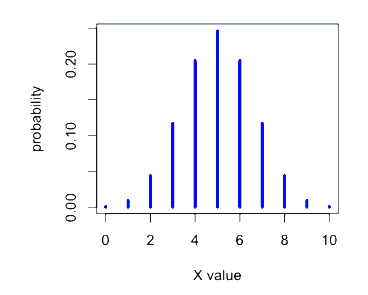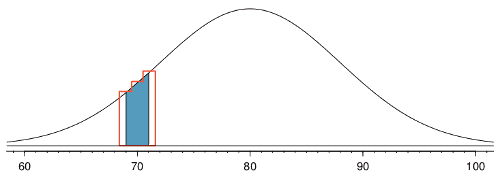# ML Wiki

## Binomial Distribution

A binomial distribution is a Discrete Distribution of Random Variables

### Intuition

Assume there are $n$ independent experiments

• an event $A$ can either appear with probability $p$ or not appear with probability $q = 1 -p$
• an RV $X$ is the number of experiments in which $A$ appeared
• such experiments are called "Bernoulli Trials"

Using Bernoulli Formula, can calculate that

• the probability of $A$ happening $k$ times out of $n$ trials is
• $P_n(k) = C_n^k \ p^k q^{n-k}$, $0 \leqslant k \leqslant n$

### Example

$X \sim \text{Bin}(10, 0.5)$

•## Main Properties

### Expected Value

$E[X] = np$

• let $X$ be the # of independent experiments in which $A$ appeared
• $X = X_1 + X_2 + ... + X_n$,
• where $X_i = 1$ if $A$ happened in experiment $i$, and $X_i = 0$ otherwise
• $E[X_i] = 1^2 \cdot p + 0^2 \cdot q = P(A)$
• $E[X] = E \left[ \sum X_i \right] = \sum E[X_i] = np$

### Variance

Thm $\text{Var}[X] = npq$

Proof

• let $X$ be the # of independent experiments in which $A$ appeared,
• $X = X_1 + X_2 + ... + X_n$
• $X_i$ are pair-wise independent, i.e. the outcome of one experiment doesn't depend on the outcome of any other experiment
• thus,
• $\text{Var}[X] = \sum_{i = 1}^n \text{Var}(X_i)$
• $E[X_i] = P(A)$
• $\text{Var}[X_i] = E[X_i^2] - E^2[X_i] = p - p^2 = p(1 - p) = pq$
• since there are $n$ $\text{Var}[X_i]$ then we multiply it by $n$:
• $\text{Var}[X] = npq$

We say that $X$ follows the Binomial Distribution

## Examples

### Example 1

Произведено 10 независимых испытаний, в каждом из которых вероятность появления события равна 0.6. Найти дисперсию С.В. $X$ - числа появления события в этих испытаниях

• $n = 10, p = 0.6, q = 1 - 0.6 = 0.4$
• $\text{Var}[X] = npq = 10 \cdot 0.6 \cdot 0.4 = 2.4$

### Example 2

Монета брошена два раза. Написать в виде таблицы закон распределения случайной величины $X$ - число выпаданий герба.

$p = 0.5, q = 0.5$

Возможные значения: $x_0 = 0, x_1 = 1, x_2 = 2$

• $P_2(2) = C_2^2 p^2 = 0.25$
• $P_2(1) = C_2^1 pq = 0.5$
• $P_2(0) = C_2^0 q^2 = 0.25$

## Normal Approximation

The Bernoulli formula is cumbersome when $n$ is large

• in some cases, the Normal Distribution is a fast way of estimating the binomial probabilities

### Binomial Coin Experiment

• go to the applet page and select "Binomial Coin Experiment"
• set # of trials to 20 and prob of success to 0.13
• we see the theoretical shape
• the applet allows to simulate coin flips and we see that the empirical distribution approaches the theoretical
• what's the $n$ when we can obtain a unimodal and symmetric distribution?R code to produce the figure
require(animation)

p = 0.13
max.n = 30

saveGIF({
for (n in 2:130) {
x = seq(1, min(n, max.n))
fx = dbinom(x=x, size=n, prob=p)
plot(x=NULL, y=NULL, xlim=c(0, max.n), ylim=c(0, 0.2),
main=paste("binomomial distribution with n =", n),
ylab="probability", xlab="outcome", axes=F)

axis(side=1); axis(side=2)

bar.width = 0.4
par(xpd=NA)
rect(xleft=x-bar.width, xright=x+bar.width,
ybottom=0, ytop=fx, col='skyblue')
}
}, interval=0.1)


We see that around $n =$ 50-60 it becomes quite symmetric

•It's reasonable to use the Normal Distribution to approximate Binomial

• parameters: $\mu = np, \sigma = \sqrt{npq}$
• note: the sample size should be sufficiently large:
• both $n \cdot p$ and $n \cdot q$ should be at least 10
• here's the same distribution ($p=0.13$, and $n$ increasing) plus $N(np, \sqrt{npq})$R code to produce the figure
saveGIF({
for (n in 2:130) {
x = seq(1, min(n, max.n))
fx = dbinom(x=x, size=n, prob=p)

plot(x=NULL, y=NULL, xlim=c(0, max.n), ylim=c(0, 0.2),
main=paste("binomomial distribution with n =", n),
ylab="probability", xlab="outcome", axes=F)

par(xpd=FALSE)
abline(v=0:30, col='grey', lty=2)
axis(side=1); axis(side=2)

par(xpd=NA)
bar.width = 0.4
rect(xleft=x-bar.width, xright=x+bar.width,
ybottom=0, ytop=fx, col='skyblue')

fn = dnorm(x=c(-1, 0, 1, x), mean=n*p, sd=sqrt(n*p*(1-p)))
xspline(x=c(-1, 0, 1, x), y=fn, lwd=2, shape=1, border="blue")
}
}, interval=0.1)


### Example

• We want to estimate the prob of observing 59 or fewer smokers in a sample of 400
• the true proportion of smokers is $p=0.20$
• Normal approximation: $\mu = np = 80$, and $\sigma = \sqrt{npq} = 8$
• so use the normal model N(80, 8) to approximate

Compute

• $Z$ score first: $Z = \cfrac{59 - 80}{8} = -2.63$
• The corresponding left tail area is 0.0043.
• the solution with the formula (using the Binomial Distribution) is 0.0041 - approximately equal

### Small Intervals

Caution: The normal approximation may fail on small intervals

• Even when the conditions are met, the approximation can still perform poorly
• it's the case when the range of counts is small

Example:

• want to compute probabilities of observing 69, 70, 71 smokers in 400 people with $p=0.2$
• Binom: 0.0703
• Norm: 0.0476

Reason why:

•• (source: Figure 3.19 from OpenIntro)
• normal curve + ares between 69 and 71 is shaded
• outlined area - exact binomial probability
• Normal approx is too fine-grained (area for ND is too slim)
• solution in this case is to add extra areas on both sides (-+0.5 and ) - this is called "Continuity Correction" 

## Usage

A Sampling Distribution for a population mean follows this distribution